# Exercise E10.3 Circles NCERT Solutions Class 9

Go back to  'Circles'

## Chapter 10 Ex.10.3 Question 1

Draw different pairs of circles. How many points does each pair have in common? What is the maximum number of common points?

### Solution

What is known?

Pair of circles.

What is unknown?

No. of points does each pair have in common and what can be maximum common points

Reasoning:

We can draw no. of possible pairs and can observe common points in each case

Steps:

In this there are no common points.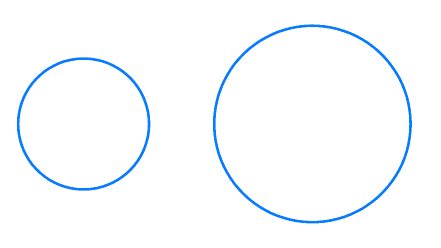In this there is only one common point.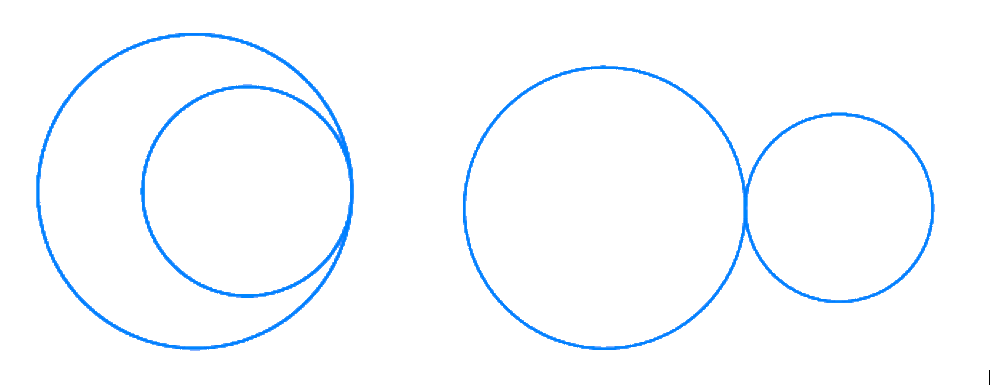In this there are two common points.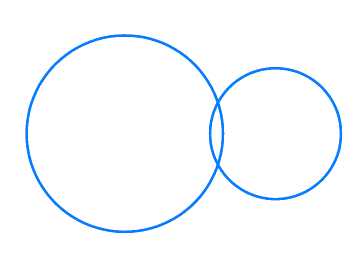In a situation where two congruent circles are superimposed on each other, it can be understood to as if we are drawing the same circle two times.

It is therefore concluded, there can be a maximum of two points for different pairs of circles.

## Chapter 10 Ex.10.3 Question 2

Suppose you are given a circle. Give a construction to find its center

### Solution

What is known?

A circle.

What is unknown?

Centre of the circle.

Reasoning:

By Theorem 10.3 (The perpendicular from the centre of a circle to a chord bisects the chord.) and by Theroem 10.4 (The line drawn through the centre of a circle to bisect a chord is perpendicular to the chord), we see that if a perpendicular bisector is drawn for a chord, it passes through the center. As it is not possible to find the center with the help of only one chord, two chords can be drawn. The intersection point of two perpendicular bisectors is the centre of the circle. Because the centre of the circle should lie on both the perpendicular bisectors of the two chords.

Steps: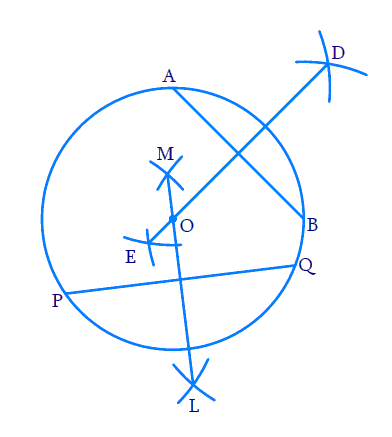Construction:

Step 1: Draw a circle with convenient radius.

Step 2: Draw $$2$$ chords \begin{align}{AB} \end{align} and \begin{align}{PQ} \end{align} of any length.

Step 3: With \begin{align}{A} \end{align} as center and radii as more than half the length of \begin{align}{AB} \end{align}, draw two arcs on opposite sides of chord \begin{align}{AB} \end{align}. With the same radius and with \begin{align}{B} \end{align} as center, draw two arcs cutting the former arcs. Join the line. Now \begin{align}{DE} \end{align} is the perpendicular bisector of \begin{align}{AB} \end{align}. Centre lies on \begin{align}{DE} \end{align}.

Step 4: With $${P}$$ as center and radii as more than half the length of \begin{align}{PQ,} \end{align} draw two arcs on opposite sides of chord \begin{align}{PQ.} \end{align} With the same radius and with \begin{align}{Q,} \end{align} as center, draw two arcs cutting the former arcs. Join the line. Now $${LM}$$ is the perpendicular bisector of $${PQ}$$. Centre lies on $${LM}$$ also.

Step 5: As the center of the circle should lie both on $${DE}$$ and $${LM},$$ it is obvious that the intersection points of $${DE}$$ and $${LM}$$ is the center. Mark the intersection points as $${O}$$.

Step 6: $${O}$$ is the required centre of the circle.

## Chapter 10 Ex.10.3 Question 3

If two circles intersect at two points, prove that their centers lie on the perpendicular bisector of the common chord.

### Solution

What is known?

Two circles intersecting at two points.

What is unknown?

To check whether their centers lie on the perpendicular bisector of the common chord.

Reasoning:

By theorem $$10.4$$, it is known that perpendicular bisector of a chord passes through its centre. Since the chord is common to both the circles, the centre should lie on the perpendicular bisector of the chord.

Steps: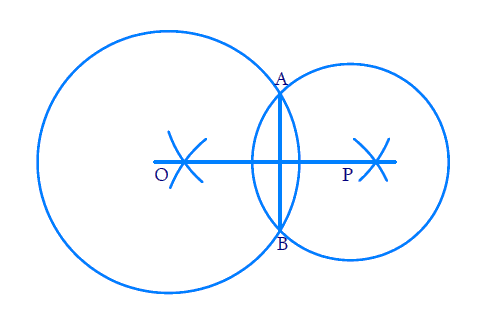Let the two circles intersect at point $${A}$$ and $${B}$$. Join $${AB}$$. $${AB}$$ is the common chord. By theorem $${10.4,}$$ it is known that perpendicular bisector of a chord passes through its centre.

As the chord $$AB$$ is common to both circles, the perpendicular bisector of the common chord should pass through their centers. So centre lies on the perpendicular bisector of the common chords.

Related Sections
Related Sections
Instant doubt clearing with Cuemath Advanced Math Program